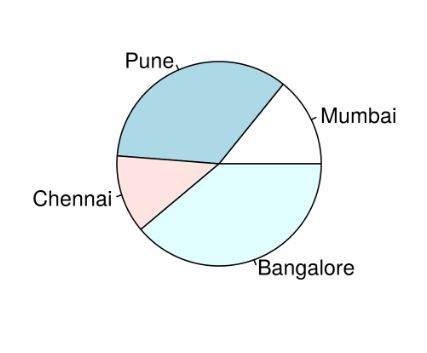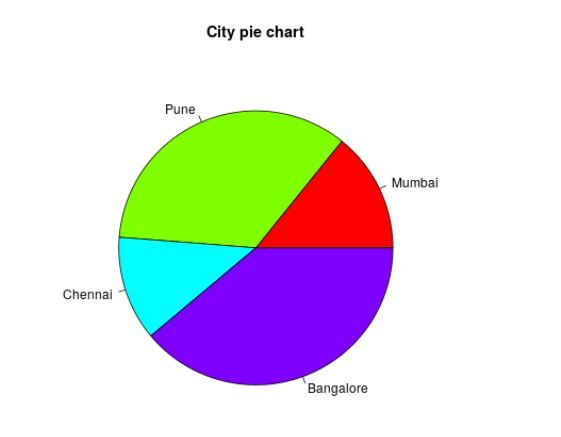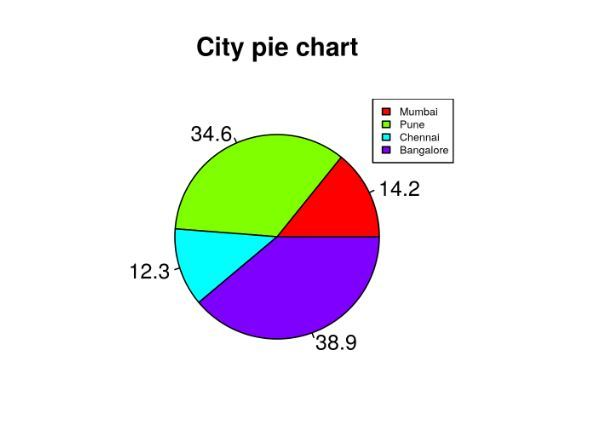# R – Pie Charts

A pie chart is a circular statistical graphic, which is divided into slices to illustrate numerical proportions. It depicts a special chart that uses “pie slices”, where each sector shows the relative sizes of data. A circular chart cuts in a form of radii into segments describing relative frequencies or magnitude also known as circle graph.

R uses the function pie() to create pie charts. It takes positive numbers as a vector input.

Syntax:

`pie(x, labels, radius, main, col, clockwise)`

Parameters:

• x: This parameter is a vector that contains the numeric values which are used in the pie chart.
• labels: This parameter gives the description to the slices in pie chart.
• radius: This parameter is used to indicate the radius of the circle of the pie chart.(value between -1 and +1).
• main: This parameter is represents title of the pie chart.
• clockwise: This parameter contains the logical value which indicates whether the slices are drawn clockwise or in anti clockwise direction.
• col: This parameter give colors to the pie in the graph.

### Creating a simple pie chart

Approach: To create a simple pie chart:

1. By using the above parameters, we can draw a pie chart.
2. It can be described by giving simple labels.

Example:

 `# Create data for the graph. ` `geeks<``-` `c(``23``, ``56``, ``20``, ``63``) ` `labels <``-` `c(``"Mumbai"``, ``"Pune"``, ``"Chennai"``, ``"Bangalore"``) ` ` `  `# Plot the chart. ` `pie(geeks, labels) `

Output:### Pie chart including the title and colors

Approach: To create color and title pie chart.

1. Take all parameters which are required to make pie chart by giving a title to the chart and add labels.
2. We can add more features by adding more parameters with more colors to the points.

Example:

 `# Create data for the graph. ` `geeks<``-` `c(``23``, ``56``, ``20``, ``63``) ` `labels <``-` `c(``"Mumbai"``, ``"Pune"``, ``"Chennai"``, ``"Bangalore"``) ` `  `  `# Plot the chart with title and rainbow color pallet. ` `pie(geeks, labels, main ``=` `"City pie chart"``,  ` `              ``col ``=` `rainbow(length(geeks))) `

Output:### Slice Percentage & Chart Legend

Approach: To create chart legend and slice percentage, we can plot by doing below methods.

1. There are two more properties of the pie chart:
1. slice percentage
2. chart legend.
2. We can show the chart in the form of percentage as well as add legends.

Example:

 `# Create data for the graph. ` `geeks <``-`  `c(``23``, ``56``, ``20``, ``63``) ` `labels <``-`  `c(``"Mumbai"``, ``"Pune"``, ``"Chennai"``, ``"Bangalore"``) ` ` `  `piepercent<``-` `round``(``100` `*` `geeks ``/` `sum``(geeks), ``1``) ` ` `  `# Plot the chart. ` `pie(geeks, labels ``=` `piepercent, ` `    ``main ``=` `"City pie chart"``, col ``=` `rainbow(length(geeks))) ` `legend(``"topright"``, c(``"Mumbai"``, ``"Pune"``, ``"Chennai"``, ``"Bangalore"``),  ` `                     ``cex ``=` `0.5``, fill ``=` `rainbow(length(geeks))) `

Output:My Personal Notes arrow_drop_upIf you like GeeksforGeeks and would like to contribute, you can also write an article using contribute.geeksforgeeks.org or mail your article to contribute@geeksforgeeks.org. See your article appearing on the GeeksforGeeks main page and help other Geeks.

Please Improve this article if you find anything incorrect by clicking on the "Improve Article" button below.

Improved By : Akanksha_Rai

Article Tags :

1

Please write to us at contribute@geeksforgeeks.org to report any issue with the above content.